# wtmm

Wavelet transform modulus maxima

## Syntax

``hexp = wtmm(x)``
``````[hexp,tauq] = wtmm(x) ``````
``[___] = wtmm(x,'MinRegressionScale',scale)``
``````[hexp,tauq,structfunc] = wtmm(___)``````
`````` [localhexp,wt,wavscales] = wtmm(x,'ScalingExponent','local')``````
``wtmm(___,'ScalingExponent','local')``
``[___] = wtmm(___,Name,Value)``

## Description

example

````hexp = wtmm(x)` returns an estimate of the global Holder exponent, `hexp`, for the real-valued, 1-D input signal, `x`. The global and local Holder exponents are estimated for the linearly-spaced moments of the structure functions from –2 to +2 in 0.1 increments.```

example

``````[hexp,tauq] = wtmm(x) ``` also returns an estimate of the partition function scaling exponents, `tauq`. ```
````[___] = wtmm(x,'MinRegressionScale',scale)` uses only scales greater than or equal to `scale` to estimate the global Holder exponent. This syntax can include any of the output arguments used in previous syntaxes.```

example

``````[hexp,tauq,structfunc] = wtmm(___)``` also returns the multiresolution structure functions, `structfunc`, for the global Holder exponent estimate. This syntax can include any of the input arguments used in previous syntaxes.```
`````` [localhexp,wt,wavscales] = wtmm(x,'ScalingExponent','local')``` returns the local Holder exponent estimates, the continuous wavelet transform `wt`, and the scales, `wavscales`, which are used to calculate the CWT used in the `wtmm` algorithm. The wavelet used in the CWT is the second derivative of a Gaussian.```

example

````wtmm(___,'ScalingExponent','local')` with no output arguments plots the wavelet maxima lines in the current figure. Estimates of the local Holder exponents are displayed in a table to the right of the plot.```
````[___] = wtmm(___,Name,Value)` returns the Holder exponent and other specified outputs with additional options specified by one or more `Name,Value` pair arguments.```

## Examples

collapse all

Estimate the global Holder exponent for Brownian motion. This monofractal signal has a Holder exponent of approximately 0.5.

```rng(100); x = cumsum(randn(2^15,1)); hexp = wtmm(x)```
```hexp = 0.5010 ```

Confirm that for a monofractal signal, the scaling exponents are a linear function of the moments. For multifractal signals, the exponents are a nonlinear function of the moments.

Load a signal that contains two time series, each with 8000 samples. `Ts1` is a multifractal signal and `Ts2` is a monofractal fractional Brownian signal. Obtain the exponents using `wtmm`.

```load RWdata; [hexp1,tauq1] = wtmm(Ts1); [hexp2,tauq2] = wtmm(Ts2);```

Plot the scaling exponents.

```expplot = plot(-2:0.1:2,tauq2,'b-o',-2:0.1:2,tauq1,'r-^'); grid on; expplot(1).MarkerFaceColor = 'b'; expplot(2).MarkerFaceColor = 'r'; legend('Ts2-Monofractal','Ts1-Multifractal','Location','SouthEast'); title('Monofractal vs. Multifractal Scaling Exponents'); xlabel('Qth Moment'); ylabel('Scaling Exponents');```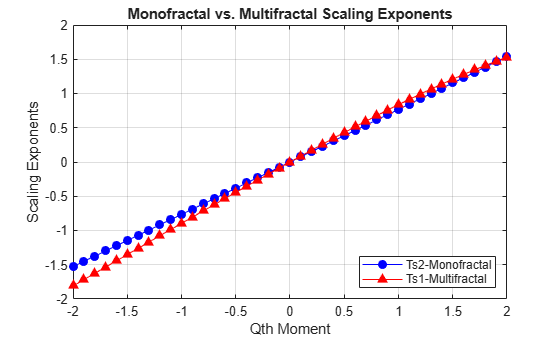`Ts2`, which is the monofractal signal, is a linear function. `Ts1`, the multifractal signal, is not linear.

Use the structure function output of `wtmm` to analyze a Brownian motion signal.

Create fractional Brownian motion with a Holder exponent of 0.6.

```Brn = wfbm(0.6,2^15); [hexp,tauq,structfunc] = wtmm(Brn);```

Compare the calculated Holder exponent with the theoretical value of 0.6.

`hexp`
```hexp = 0.6072 ```

Use the data in the `structfunc` output and the `lscov` function to perform the regression on the data.

```x = ones(length(structfunc.logscales),2); x(:,2) = structfunc.logscales; betahat = lscov(x,structfunc.Tq,structfunc.weights); betahat = betahat(2,:);```

Plot and compare the scaling exponents from the `tauq` output and from the regressed structure function output.

```subplot(1,2,1) plot(-2:.1:2,tauq) grid on title('From tauq Output') xlabel('Qth Moment') ylabel('Scaling Exponents') subplot(1,2,2) plot(-2:.1:2,betahat(1:41)) grid on title('From structfunc Output') xlabel('Qth Moment')```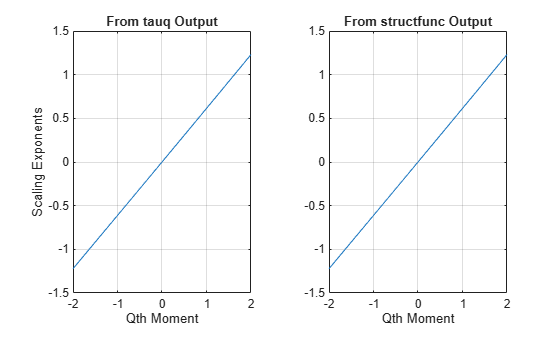The plots are the same and show a linear relationship between the moments and the exponents. Therefore, the signal is monofractal. The Holder exponent returned in `hexp` is the slope of this line.

Using a cusp signal and a signal containing delta functions, generate their local Holder exponents.

Cusp Signal

Load and plot a cusp signal. Note the difference between the two cusps.

```load cusp; plot(cusp) grid on xlabel('Sample') ylabel('Amplitude')```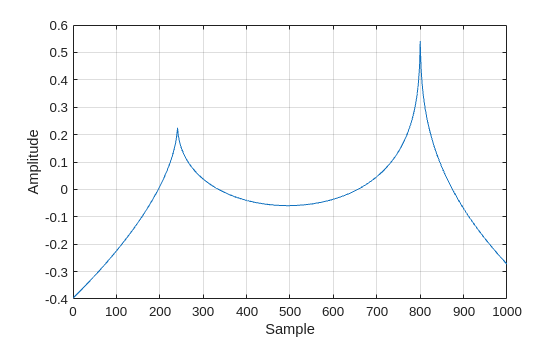The equation for this cusp signal specifies a Holder exponent of 0.5 at sample 241 and a Holder exponent of 0.3 at sample 803.

`-0.2*abs(x-241)^0.5 - 0.5*abs(x-803)^0.3 + 0.00346*x + 1.34`

Obtain the local Holder exponents and plot the modulus maxima.

`wtmm(cusp,'ScalingExponent','local');`The Holder exponents at samples 241 and 803 are very close to the values specified in the cusp signal equation. The higher Holder value at sample 241 indicates that the signal at that point is closer to being differentiable than the signal at sample 803, which has a smaller Holder value.

Delta Functions

Create and plot two delta functions.

```x = zeros(1e3,1); x([200 500]) = 1; plot(x) grid on xlabel('Sample') ylabel('Amplitude')```Obtain the local Holder exponents using the default number of octaves, which in this case is 7. Plot the modulus maxima. A delta function has a Holder exponent of -1.

`wtmm(x,'ScalingExponent','local');`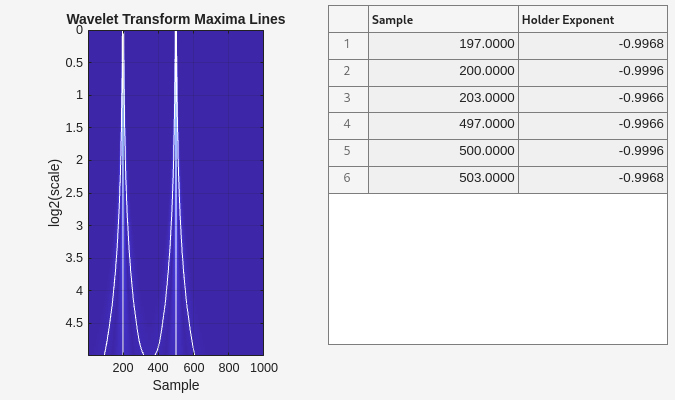Obtain the local Holder exponents using 5 octaves and compare the modulus maxima plot to the plot using the default number of octaves.

`wtmm(x,'ScalingExponent','local','NumOctaves',5);`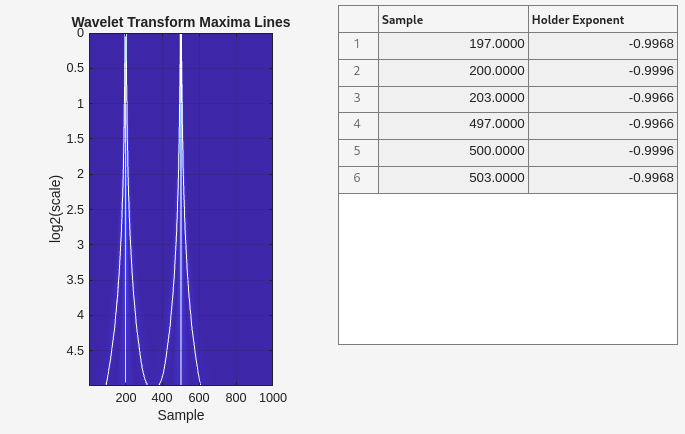Reducing the number of scales provides more separation in frequency and less overlap between the modulus maxima lines of the delta functions.

## Input Arguments

collapse all

Input signal, specified as a real-valued vector with a minimum of 128 samples. The wavelet transform modulus maxima technique works best for data with 8000 or more samples.

### Name-Value Arguments

Specify optional pairs of arguments as `Name1=Value1,...,NameN=ValueN`, where `Name` is the argument name and `Value` is the corresponding value. Name-value arguments must appear after other arguments, but the order of the pairs does not matter.

Before R2021a, use commas to separate each name and value, and enclose `Name` in quotes.

Example: `'VoicesPerOctave',18` estimates the global Holder estimate using 18 voices per octave.

Minimum scale for regression, specified as the comma-separated pair consisting of `'MinRegressionScale'` and a scalar greater than or equal to 4. This scale is the smallest scale used by the regression. There must be at least two scales with more than 6 CWT maxima. `'MinRegressionScale'` applies only to global Holder exponents.

Number of voices per octave, specified as the comma-separated pair consisting of `'VoicesPerOctave'` and an even integer from 8 to 32. The number of voices per octave and the number of octaves determine the number of scales used in the CWT.

Number of octaves, specified as the comma-separated pair consisting of `'NumOctaves'` and an integer. The number of octaves and the number of voices per octave determine the number of scales used in the CWT. The maximum number of octaves is less than or equal to `floor(log2(numel(x)/(3*sqrt(1.1666))))`. The `sqrt(1.1666)` factor is the standard deviation of the second derivative of a Gaussian wavelet. If you specify the number of octaves as greater than the maximum number of octaves, `wtmm` uses the maximum supported number of octaves.

Type of scaling exponents, specified as a comma-separated pair consisting of `'ScalingExponent'` and either `'global'` or `'local'`. A global Holder exponent is used for monofractal signals, such as white noise, which are singular everywhere. Global holder exponents give a single estimate of degree of these singularities over the whole signal. Local Holder exponents are useful for signals with cusp singularities.

## Output Arguments

collapse all

Global Holder exponent, returned as a real scalar. Holder exponents are useful for identifying singularities, which are locations where a signal is not differentiable. A global Holder exponent uses a single value to estimate the degree of differentiability of all of the singularities of a signal. Signals with a global Holder exponent are monofractal signals.

Scaling exponents, returned as a column vector. The exponents are estimated for the linearly-spaced moments of the structure functions from –2 to +2 in 0.1 increments.

Multiresolution structure functions for the global Holder exponent estimates, returned as a `struct`. The structure function for data `x` is defined as

`$S\left(q,a\right)=\frac{1}{{n}_{a}}\sum _{k=1}^{{n}_{a}}{|{T}_{x}\left(a,k\right)|}^{q}\simeq {a}^{\zeta \left(q\right)},$`

where a is the scale, q is the moment, Tx is the maxima at each scale, na is the number of maxima at each scale, and $\zeta \left(q\right)$ is the scaling exponent. `structfunc` is a structure array containing the following fields:

• `Tq` — Measurements of the input, `x`, at various scales. `Tq` is a matrix of multiresolution quantities that depend jointly on time and scale. Scaling phenomena in `x` imply a power-law relationship between the moments of `Tq` and the scale. `Tq` is an Ns-by-44 matrix, where Ns is the number of scales. The first 41 columns of `Tq` contain the scaling exponent estimates for each of the qth from -2:0.1:2, by scale. The last three columns correspond to the first-order, second-order, and third-order cumulants, respectively, by scale. For a monofractal signal, cumulants greater than the first cumulant are zero.

• `weights` — Weights used in the regression estimates. The weights correspond to the number of wavelet maxima at each scale. `weights` is an Ns-by-1 vector.

• `logscales` — Scales used as predictors in the regression. `logscales` is an Ns-by-1 vector with the base-2 logarithm of the scales.

Local Holder exponent estimates, returned as an M-by-2 array of real values, where M is the number of maxima. If no maxima lines converge to the finest scale in the wavelet transform, then `localhexp` is an empty array. The wavelet transform modulus maxima method (WTMM) identifies cusp-like singularities in a signal. To analyze multifractal signals, use `dwtleader`.

Continuous wavelet transform, returned as a matrix of real values. `wt` is a `numel(wavscales)-by-N` matrix where `N` is the length of the input signal `x`.

Wavelet scales, returned as a column vector of real values. `wavscales` are the scales used to calculate the CWT.

## Algorithms

The WTMM algorithm finds singularities in a signal by determining maxima. The algorithm first calculates the continuous wavelet transform using the second derivative of a Gaussian wavelet with 10 voices per octave. The wavelet that meets this criteria is the Mexican hat, or Ricker, wavelet. Then, the algorithm determines the modulus maxima for each scale. The WTMM is intended to be used with large data sets so that enough samples are available to determine maxima accurately.

The definition of the modulus maximum at point x0 and scale s0 is

`$|Wf\left({s}_{0},x\right)|<|Wf\left({s}_{0},{x}_{0}\right)|$`

where x is either in the right or left neighborhood of x0. When x is in the opposite neighborhood of x0, the definition is

`$|Wf\left({s}_{0},x\right)|\le |Wf\left({s}_{0},{x}_{0}\right)|$`

. The algorithm for finding additional maxima repeats for values in that scale. Then, the algorithm continues up through finer scales, checking whether the maxima align between scales. If a maximum converges to the finest scale, it is a true maximum and indicates a singularity at that point.

When each singularity is determined, the algorithm then estimates its Holder exponent. Holder exponents indicate the degree of differentiability for each singularity, which classifies the singularity strength. A Holder exponent less than or equal to 0 indicates a discontinuity at that location. Holder exponents greater than or equal to 1 indicate that the signal is differentiable at that location. Holder values between 0 and 1 indicate continuous, but not differentiable locations. They indicate how close the signal at that sample is to being differentiable. Holder exponents close to 0 indicate signal locations that are less differentiable than locations with exponents closer to 1. The signal is smoother at locations with higher local Holder exponents.

For signals with a few cusp-like singularities and Holder exponents that have large variation, you set the algorithm to return local Holder exponents, which provide individual values for each singularity. For signals with numerous Holder exponents that have relatively small variations, you set the algorithm to return a global Holder exponent. A global Holder exponent applies to the whole signal. For signals with many singularities, you can reduce the number of maxima found by limiting the algorithm to start at or regress to a specific minimum or maximum scale, respectively. For detailed information about the WTMM, see  and .

 Mallat, S., and W. L. Hwang. “Singularity Detection and Processing with Wavelets.” IEEE Transactions on Information Theory. Vol. 38, No. 2, March 1992, pp. 617–643.

 Wendt, H. and P. Abry. “Multifractality Tests Using Bootstrapped Wavelet Leaders.” IEEE Transactions on. Signal Processing. Vol. 55, No. 10, 2007, pp. 4811–4820.

 Arneodo, A., B. Audit, N. Decoster, J.-F. Muzy, and C. Vaillant. “Wavelet-Based Multifractal Formalism: Application to DNA Sequences, Satellite Images of the Cloud Structure and Stock Market Data.” The Science of Disasters: Climate Disruptions, Heart Attacks, and Market Crashes. Bunde, A., J. Kropp, and H. J. Schellnhuber, Eds. 2002, pp. 26–102.